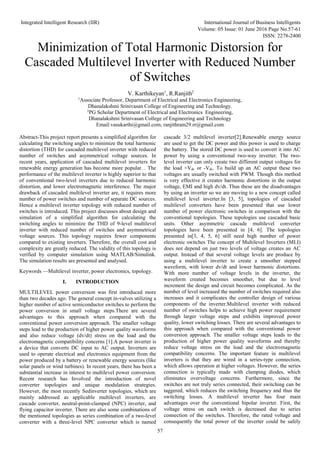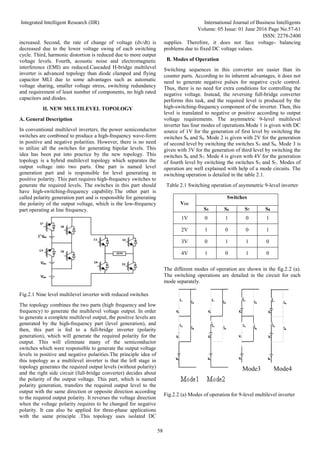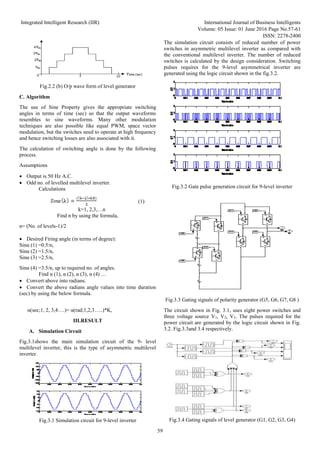Seu SlideShare está sendo baixado. ×

# Minimization of Total Harmonic Distorsion for Cascaded Multilevel Inverter with Reduced Number of Switches

Anúncio
Anúncio
Anúncio
Anúncio
Anúncio
Anúncio
Anúncio
Anúncio
Anúncio
Anúncio
AnúncioAnúncio
Carregando em…3
×

1 de 5 Anúncio

# Minimization of Total Harmonic Distorsion for Cascaded Multilevel Inverter with Reduced Number of Switches

This project report presents a simplified algorithm for calculating the switching angles to minimize the total harmonic distortion (THD) for cascaded multilevel inverter with reduced number of switches and asymmetrical voltage sources. In recent years, application of cascaded multilevel inverters for renewable energy generation has become more popular. . The performance of the multilevel inverter is highly superior to that of conventional two-level inverters due to reduced harmonic distortion, and lower electromagnetic interference. The major drawback of cascaded multilevel inverter are, it requires more number of power switches and number of separate DC sources. Hence a multilevel inverter topology with reduced number of switches is introduced. This project discusses about design and simulation of a simplified algorithm for calculating the switching angles to minimize the THD of 9-level multilevel inverter with reduced number of switches and asymmetrical voltage sources. This topology requires fewer components compared to existing inverters. Therefore, the overall cost and complexity are greatly reduced. The validity of this topology is verified by computer simulation using MATLAB/Simulink. The simulation results are presented and analysed.

This project report presents a simplified algorithm for calculating the switching angles to minimize the total harmonic distortion (THD) for cascaded multilevel inverter with reduced number of switches and asymmetrical voltage sources. In recent years, application of cascaded multilevel inverters for renewable energy generation has become more popular. . The performance of the multilevel inverter is highly superior to that of conventional two-level inverters due to reduced harmonic distortion, and lower electromagnetic interference. The major drawback of cascaded multilevel inverter are, it requires more number of power switches and number of separate DC sources. Hence a multilevel inverter topology with reduced number of switches is introduced. This project discusses about design and simulation of a simplified algorithm for calculating the switching angles to minimize the THD of 9-level multilevel inverter with reduced number of switches and asymmetrical voltage sources. This topology requires fewer components compared to existing inverters. Therefore, the overall cost and complexity are greatly reduced. The validity of this topology is verified by computer simulation using MATLAB/Simulink. The simulation results are presented and analysed.

Anúncio
Anúncio